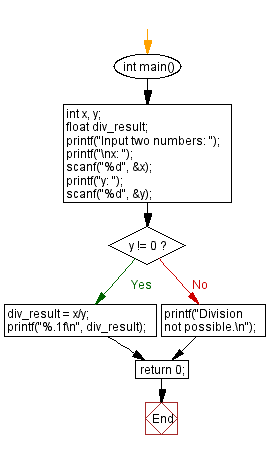﻿ C : Read two numbers and divide two numbers by each other

# C Exercises: Read two numbers and divide the first number by second number

## C Basic Declarations and Expressions: Exercise-38 with Solution

Write a program that reads two numbers and divides the first number by the second number. If division is not possible print "Division is not possible".

Sample Solution:

C Code:

``````#include <stdio.h>
int main() {
int x, y;
float div_result;

// Prompt for user input
printf("Input two numbers: ");
printf("\nx: ");
scanf("%d", &x);
printf("y: ");
scanf("%d", &y);

// Check if division is possible
if(y != 0) {
div_result = x/y;
printf("%.1f\n", div_result);
} else {
printf("Division not possible.\n");
}

return 0;
}
``````

Sample Output:

```Input two numbers:
x: 25
y: 5
5.0
```

Flowchart:C programming Code Editor:

What is the difficulty level of this exercise?

Test your Programming skills with w3resource's quiz.

﻿